Question

# Can anyone help me prove the theorem 49 by using the following hint( Circle)? Here is...

Can anyone help me prove the theorem 49 by using the following hint( Circle)?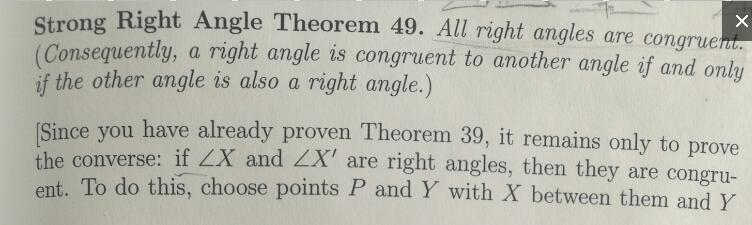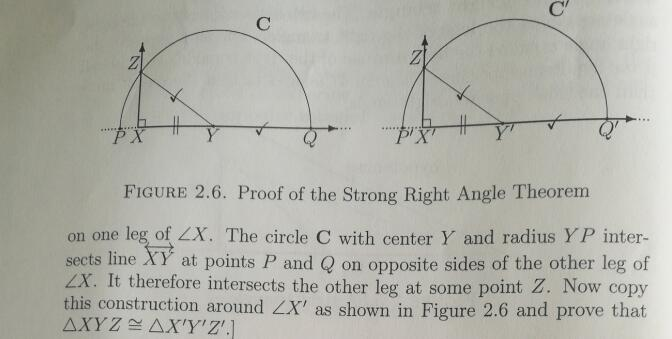Here is Theorem 39, can't use like right angles are 90 degrees, or that triangles are 180 degrees.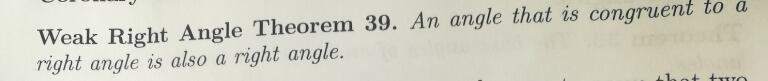Strong Right Angle Theorem 49. All right angles are congruer Consequently, a right angle is congruent to another angle if and only if the other angle is also a right angle.) Since you have already proven Theorem 39, it remains only to prove the converse: i f LX and ZX' are right angles, then they are congru- ent. To do this, choose points P and Y with X between them and Y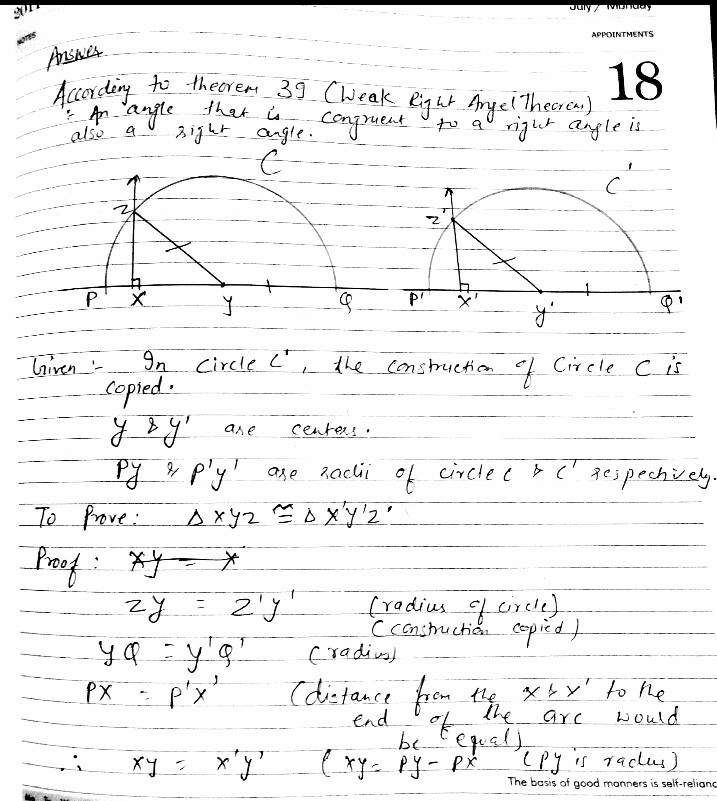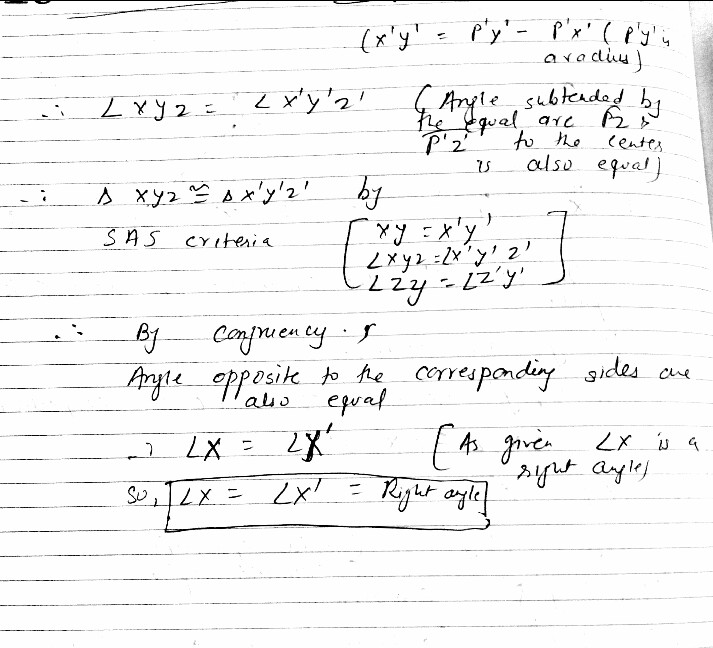#### Earn Coins

Coins can be redeemed for fabulous gifts.

Similar Homework Help Questions
• ### what is the largest prime number you will need to check to find all the prime numbers less than 100 Here is a site that I found searching Google with the key words, "<I>prime numbers</I>

what is the largest prime number you will need to check to find all the prime numbers less than 100Here is a site that I found searching Google with the key words, "prime numbers." http://mathforum.org/dr.math/faq/faq.prime.num.html I hope this helps. Thanks for asking.Finding Primes The Sieve of Eratosthenes Lets find the primes between 1 and 100. Write down the sequence of numbers from 1 to 100. Cross out the 1. Beginning with the 2, strike out every second number beyond the...